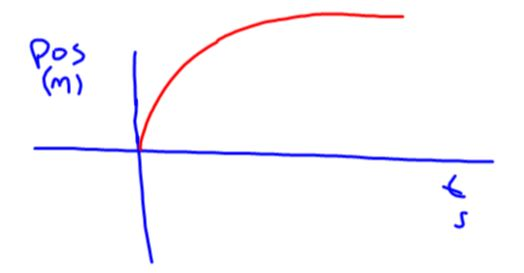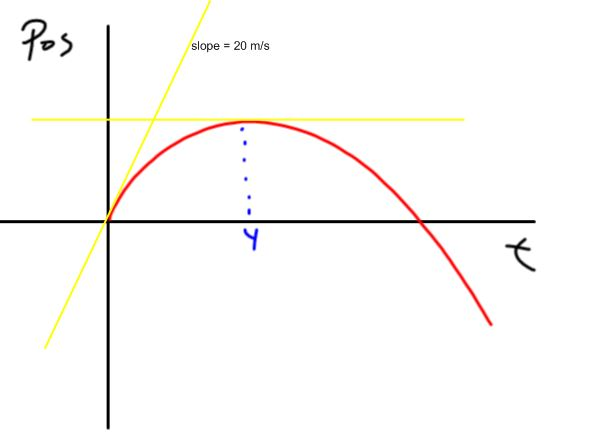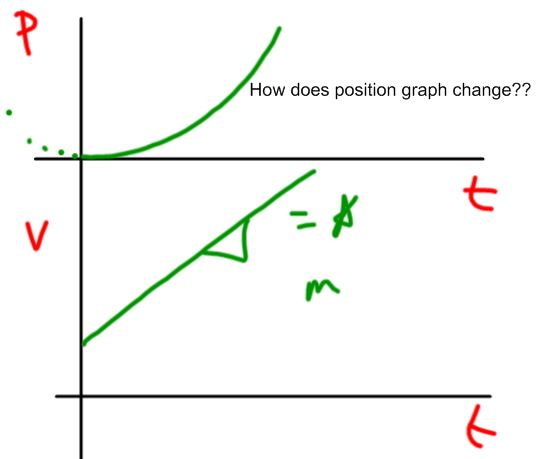# Graphical Summary of Motion

## Objective

Students will create velocity and position graphs from provided information.

#### Big Idea

The idea of slope unites an object's motion graphs.

## Overview

Today's lesson is truncated to just 40 minutes or so. This is the day before our April break and some families have chosen to take their children out of school for early travelling. In addition, two of our winter teams won their respective State Championships and are travelling to the Vermont State House to meet the Governor and be recognized by the legislature. This is an awesome opportunity for those students but has severe implications for attendance today - my classes are significantly depleted. I wish to honor the time of the students who are in attendance but also need to recognize the reality of inevitable re-teaching.

Today's lesson allows us to develop the idea that objects in motion can be modeled graphically (Science & Engineering practice #2). It is a kind of reasoning that is non-computational, though very much mathematical (Science & Engineering practice #5). By becoming fluent with the ideas of position, velocity, and acceleration, students continue in the long progression toward the achievement of NGSS Performance Expectation HS-PS2-1.

## Warmup: Velocity Functions

10 minutes

As a way to continue our thinking about slopes and motion graphs, I ask my students to try the following velocity warmup questions. Though the wording varies from question to question, the essential idea is the same: the velocity graphs are linear with a slope that is equal to the acceleration and the y-intercept will identify the initial velocity.

I give students a few minutes to work on these problems in their notebooks. They may work individually or in small groups on these questions. The second set of questions requires some more agile thinking - creating position graphs from velocity information is much more challenging.

After about ten minutes, I open the discussion at the board. I want these problems to be the foundation of alter work and want to take time to fully explore them.

## Discussion of Velocity Function Warmups

15 minutes

I show the following drawing, corresponding to the fist four questions in the warmup set, and have students copy them, if necessary. If the central concept of acceleration being the slope of the velocity graph can be grasped, then these answers can be absorbed quickly. The answers to #3 and #4 (in red) are shown on the same axes to highlight the shared initial velocities.Before I show any solutions to the position graph questions, I ask a student to put her sketch of the first of these questions on the board. She produces the following sketchThere is much to celebrate here. She has correctly identiifed the non-linear nature of the position graph. She has also started the sketch with an upswing - the slope of a would be tangent line at zero seconds should be positive. Furthermore, she has the curvature of the function essentially correct - the curve flattens over time. What she failed to include is the idea that, once it truly flattens, any would-be tangent line would, from thereafter, be negative. We discuss all of these ideas, then reveal a more complete answerAt the end of this discussion, students are ready for a more generalized set of ideas about motion graphs. This is provided in the next segment of class.

## Graphical Summary of Motion

15 minutes

I share the following triple-graph with students as they copy this into their notebooks. It is entitled "constant velocity" and shows the relationship between the position, velocity, and accelerations graphs. I use the "heart" symbol as a way to generalize the relationship between the slope of the position graph and the resulting velocity.After some clarifying thoughts, I reveal the second part of this slide; the same kinds of graphs for the constant acceleration case.As a follow-up to these graphs, I ask students to commit to a sketch of a position graph if I make a change to the velocity graph as shown below, where the starting velocity is non-zero:Because the initial velocity is no longer zero, the position graph needs to shift so that the slope of a tangent line, drawn at zero seconds, would be non-zero. Indeed, the shift needs to happen so that the parabolic position graph starts on an upswingWe end the class with the promise that this kind of thinking will be featured in upcoming lessons.